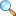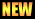Chapter 02: The Derivative

Notes of the book Calculus with Analytic Geometry written by Dr. S. M. Yusuf and Prof. Muhammad Amin, published by Ilmi Kitab Khana, Lahore - PAKISTAN.

Here are few online resource, which are very helpful to find derivative.

Contents and summary

• Definition and notation
• Geometrical interpretation of the derivative
• Instantaneous velocity
• Marginal functions in economics
• General theorems on derivatives
• Inverse trigonometric functions
• Logarithmic and exponential functions
• Derivative of hyperbolic functions
• Implicit Differentiation
• Logarithmic Differentiation
• Differentials and approximation
• Newtorn Raphson Method
• Higer derivatives
• Some standard nth derivatives
• Leibniz rule
• Functions of several variables
• Open and closed rectangles
• Limit and continuity
• Partial derivatives
• Differentiability

Solutions

• Exercise 2.4 |View online | Download PDF• bsc/notes_of_calculus_with_analytic_geometry/ch02_derivatives